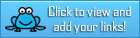# Tag Archives : #educators

## Complete Tone and Mood in Literature Bundle + Connotation and Denotation

Teach BeTween the Lines

Teach your students how to understand the complex topic of tone and mood in literature!
1. Lesson on Tone and Mood
2. Lesson on Connotation/Denotation
3. Tone and Mood Exploration through music, video games, film, art, literature, photography, and memes!
4. Tone and Mood Mini-Lesson
5. Tone and Mood Notes
6. Tone and Mood Project- over 8 choices for summative assessments!
7. Bell Ringer Activities- for each day of the unit!

Lesson on Connotation/Denotation: Students learn the impact of word choice on the author’s tone. Lesson includes a collaborative and engaging student activity to practice and apply skills! My students rave about it!

Tone and Mood Exploration: Students explore tone and mood through music, video games, film, art, literature, photography, and memes! This collaborative (or independent) activity is student driven. Differentiated worksheets support students of all levels! Students practice and apply their knowledge of tone to varying subjects and mediums. It is perfect to show students that similar subjects can take on a vary different tone given the context.

Tone and Mood Mini-Lesson: Offer struggling students extra support with this small group mini-lesson and note taking guide. Google Slides/Doc and PowerPoint/Word options provided!

Tone and Mood Summative Assessment: Offer students voice and choice with 9 different engaging and interesting summative assessment options for demonstrating knowledge of the standard. All rigorous assessment options ask students to think critically and synthesize knowledge gleaned throughout the unit.

I have recently opened my teaching style to include a personalized learning platform of teaching and learning. I have started offering more voice and choice in learning options, as well as assessment options. I have included both a standard set of lesson plans, as well as a personalized learning plan and 9 day outline of teaching, coaching, and assessing options for both models of instruction.

If your students are new to the personalized learning model, I have included a Google Slides/PowerPoint Presentation to introduce and support your learners as you shift in your teaching style.CCSS Standards:

CCSS.ELA-LITERACY.RL.7.4
CCSS.ELA-LITERACY.RL.8.4
CCSS.ELA-LITERACY.RL.9-10.4
CCSS.ELA-LITERACY.RL.11-12.4

I am happy to support you throughout this unit, offer advice, suggestions, or feedback as you work to personalize learning. Email me at teachbetweenthelinestpt@gmail.com

I also have a blog series dedicated to supporting teachers as they personalize learning- feel free to check this out for more detailed implementation ideas: So, You Have Been Asked To Personalize Learning- What Now?

Follow my store by clicking on the link at the top of the page for updates, announcements, and fun freebies! You can also find me on Pinterest, and Facebook!

## February Teacher Talk

Posted by Deann Marin of Socrates Lantern

Welcome to our February Teacher Talk.  All of us from the Teacher Talk collaborative would like wish you a Happy Valentines Day.  We have so many fab things this month from  proof-reading ideas, to ELA to math activities, to celebrating Black History Month and President’s Day, you don’t want to miss reading these blog posts from some awesome educators.If you’re interested in joining this unique group of teacher entrepreneurs and blogging buddies and our blog linky, sign up here….The Best of Teacher Entrepreneurs Marketing Cooperative. If you decide to join, be sure to mention one of our names.

<<<<<<<<<<<<<<<<<<<<<<<<<<<<<<<<<<<<<<<<<<<<<<<<<<<<<<<<<<<

The Proof is in the Pudding, Proof-Reading That Is!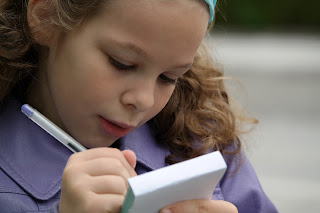As a veteran English and Social Studies teacher, I’d like to share some effective proof-reading tips that I’ve used to help students improve their writing skills, and to make my life a bit easier.

<<<<<<<<<<<<<<<<<<<<<<<<<<<<<<<<<<<<<<<<<<<<<<<<<<<<<<<<<<<

Love is All We Need

By Retta London of Rainbow City LearningJust a little inspiration for building confidence and acceptance in your learning community.

<<<<<<<<<<<<<<<<<<<<<<<<<<<<<<<<<<<<<<<<<<<<<<<<<<<<<<<<<<<

IMWAYR: Funny Bones

By Lisa Robles of LisaTeachR’s ClassroomHave you read Funny Bones? Winner of multiple awards and a great informational addition to your Dia de Los Muertos collection!

<<<<<<<<<<<<<<<<<<<<<<<<<<<<<<<<<<<<<<<<<<<<<<<<<<<<<<<<<<<

Valentine’s Day STEM Olympics

By Kerry Tracy of Kerry TracyBlog post outlines 5 infatuating Valentine’s Day-themed STEM challenges that can be modified for use with grades 2-8. Help Cupid get some target practice, build a tower of love, design the perfect candy container, find the “heaviest” heart, and have a flower frenzy!

<<<<<<<<<<<<<<<<<<<<<<<<<<<<<<<<<<<<<<<<<<<<<<<<<<<<<<<<<<<

Cooperative Learning – Bring Core Subjects Together for Student Learning!

By M. Moore of Moore ResourcesCooperative Learning – Bring Core Subjects Together for Student Learning!

<<<<<<<<<<<<<<<<<<<<<<<<<<<<<<<<<<<<<<<<<<<<<<<<<<<<<<<<<<<

What Word Does This Say: B-L-E-N-D?

By Susan Berkowitz of Susan BerkowitzThis is part of a series of posts about phonological awareness and the different steps and skills to teach. This post is about blending sounds and syllables to hear the word.

<<<<<<<<<<<<<<<<<<<<<<<<<<<<<<<<<<<<<<<<<<<<<<<<<<<<<<<<<<<

Teaching Self-Regulation Skills to Elementary Age Children

By Thia Triggs of Print PathFinally! A systematic method to teach self-regulation skills to children with sensory, emotional, and behavioral needs. Color coded task cards give students managed choices to learn specific skills that meet their sensory needs and also calm them down, so that they can perform their academic classroom tasks.

<<<<<<<<<<<<<<<<<<<<<<<<<<<<<<<<<<<<<<<<<<<<<<<<<<<<<<<<<<<

My Funny Valentine: Love Letters by Arnold Adoff

By Tracy Willis of Wild Child Designs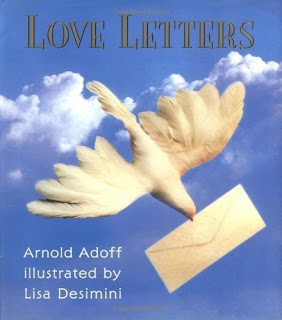A review and glimpse into “Love Letters” poetry by Arnold Adoff. This post also includes a teaching idea for its use in the classroom.

<<<<<<<<<<<<<<<<<<<<<<<<<<<<<<<<<<<<<<<<<<<<<<<<<<<<<<<<<<<

Robots + Math & Science = Total Engagement

By Megan Bodmann of Adventures Teaching 4thGet your students engaged in learning by introducing robots into your classroom. They are not only a ton of fun, but you can easily utilize them in your math and science lessons. Find out how easy it is to do!

<<<<<<<<<<<<<<<<<<<<<<<<<<<<<<<<<<<<<<<<<<<<<<<<<<<<<<<<<<<

Playground Problem = Real Life

By Kathie Yonemura of Tried and True Teaching Tools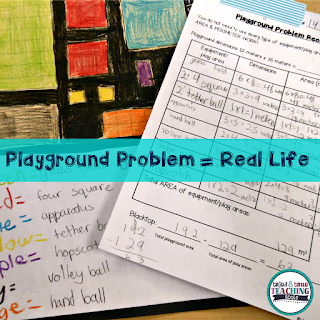The Playground Problem is a real-life math challenge! It keep students engaged and practicing their area and perimeter skills in a meaningful way.

<<<<<<<<<<<<<<<<<<<<<<<<<<<<<<<<<<<<<<<<<<<<<<<<<<<<<<<<<<<

Understanding Fractions: A 6-Part Series

By Shametria Routt of The Routty Math TeacherFractions– a single word that deflates the confidence of our most competent students and adults alike. In this series, I share some of the essential fraction understandings that I have developed over the years, including the many math tools you can use to reinforce these essential skills. With that in mind, each of the six posts highlights a fraction tool and activity that can be used to address a specific Common Core Math Standard (with a few printable freebies too).

<<<<<<<<<<<<<<<<<<<<<<<<<<<<<<<<<<<<<<<<<<<<<<<<<<<<<<<<<<<

Leveled Math Assessments

By Tammy Roose of Tarheel State Teacher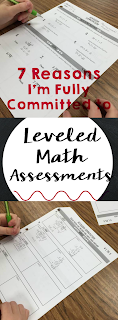Do your math assessments encourage a growth mindset? Create an entry point for students who are not yet meeting the standards? Allow above average learners to show they know more than just what’s expected for their grade-level? Find out 7 reasons why I’m committed to leveled math assessments this year!

<<<<<<<<<<<<<<<<<<<<<<<<<<<<<<<<<<<<<<<<<<<<<<<<<<<<<<<<<<<

Here’s your chance to hop on over and visit the blog posts of our creative teachers.